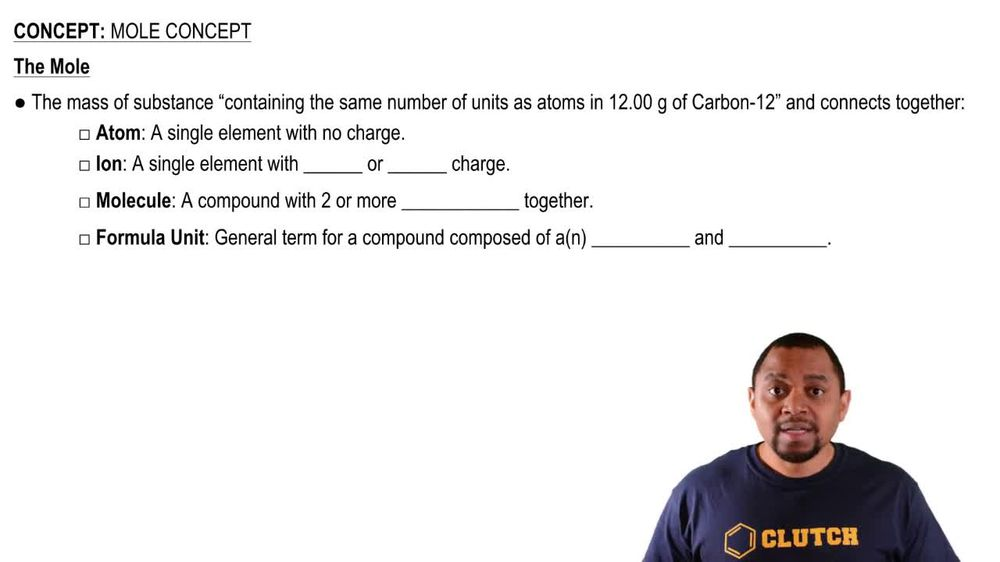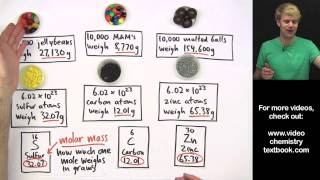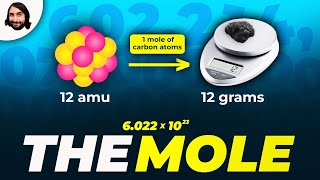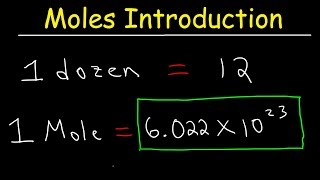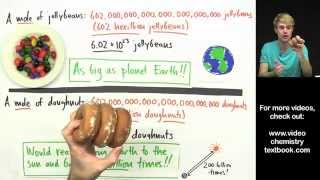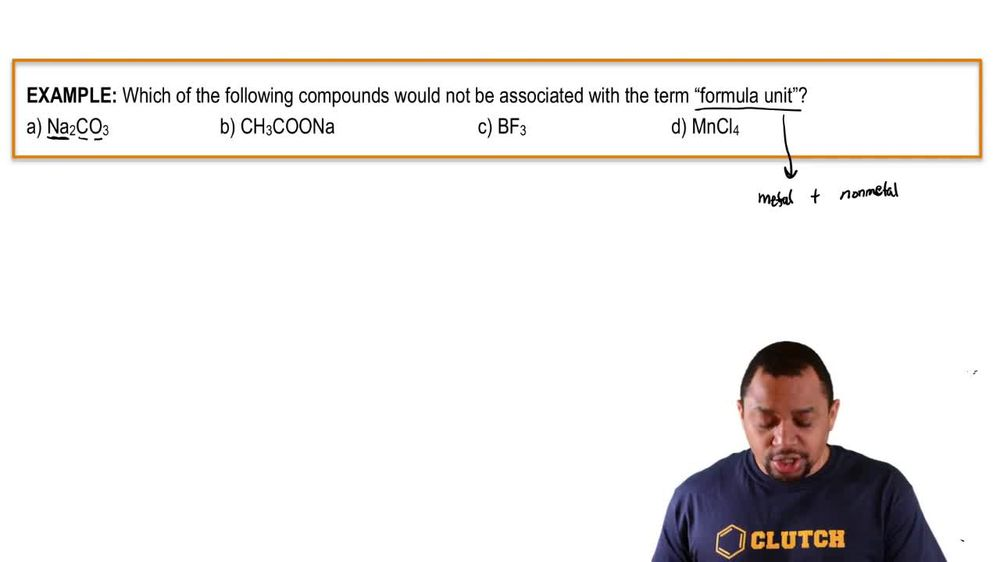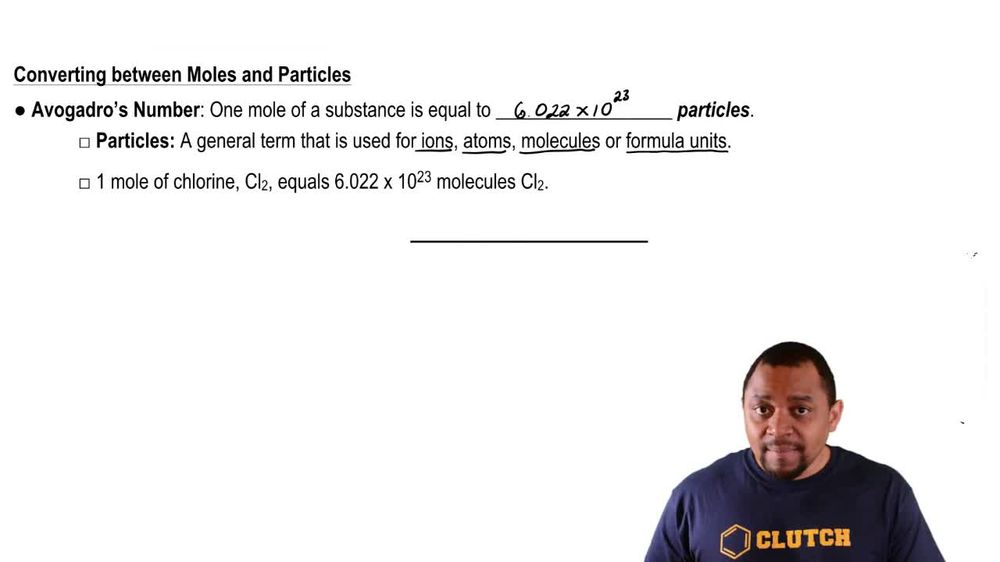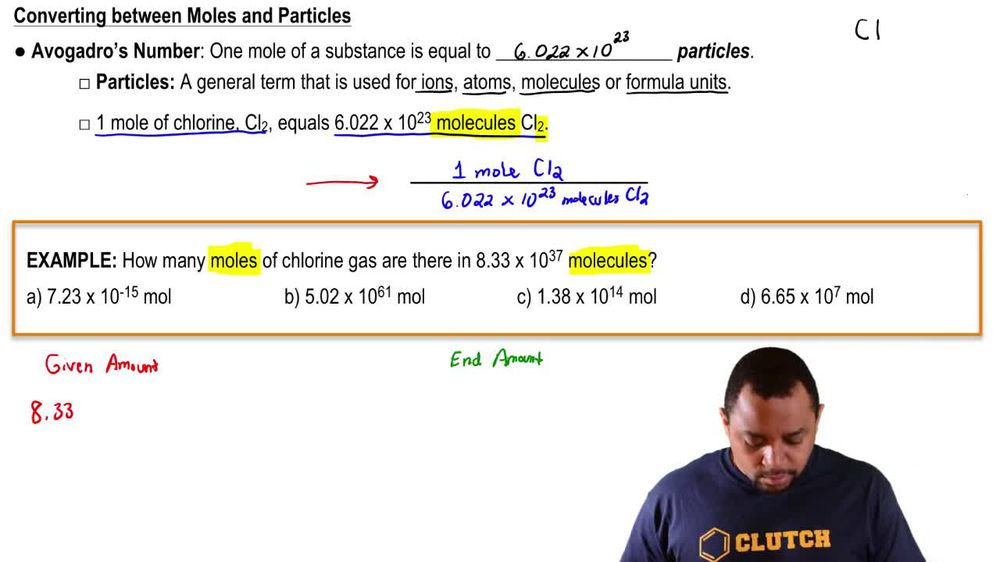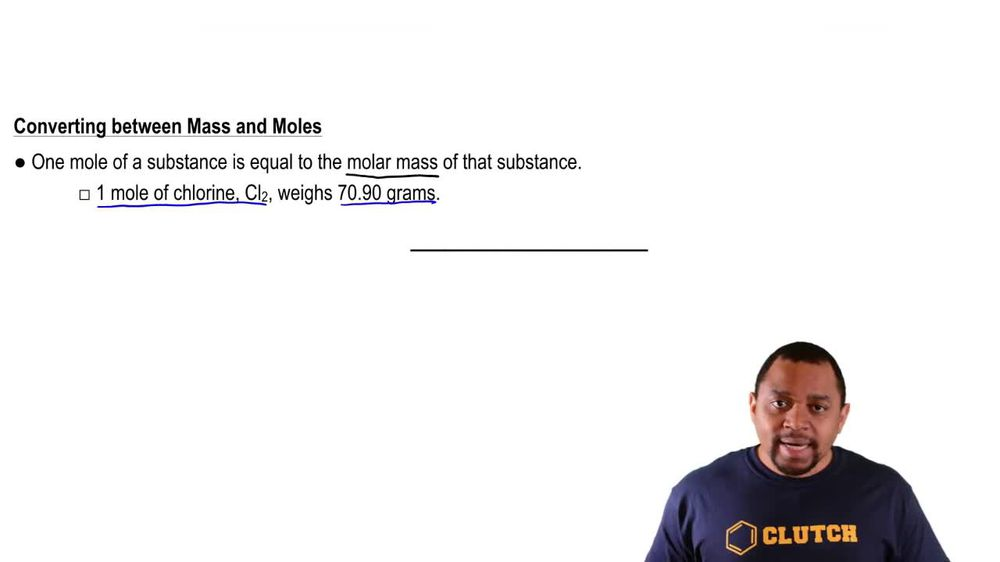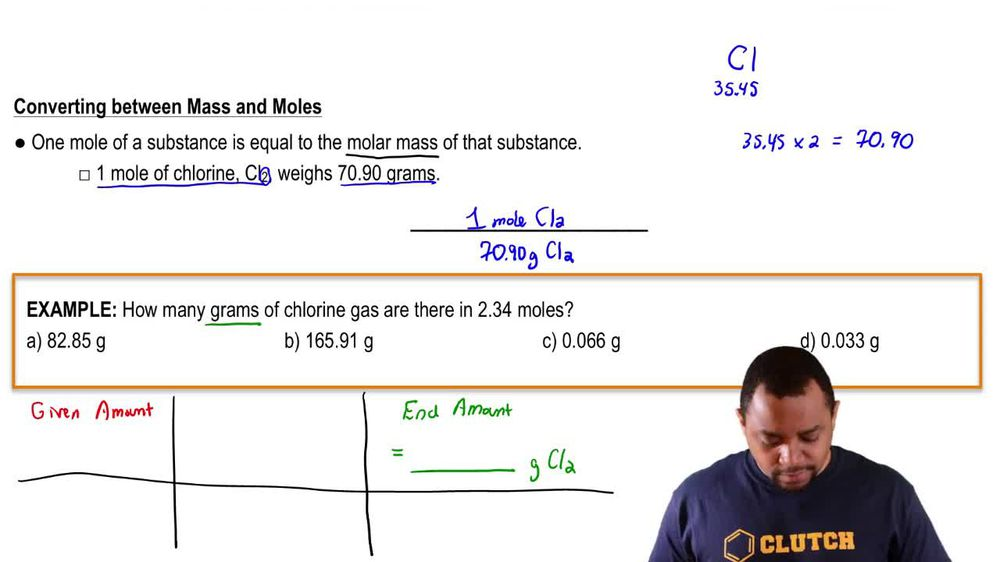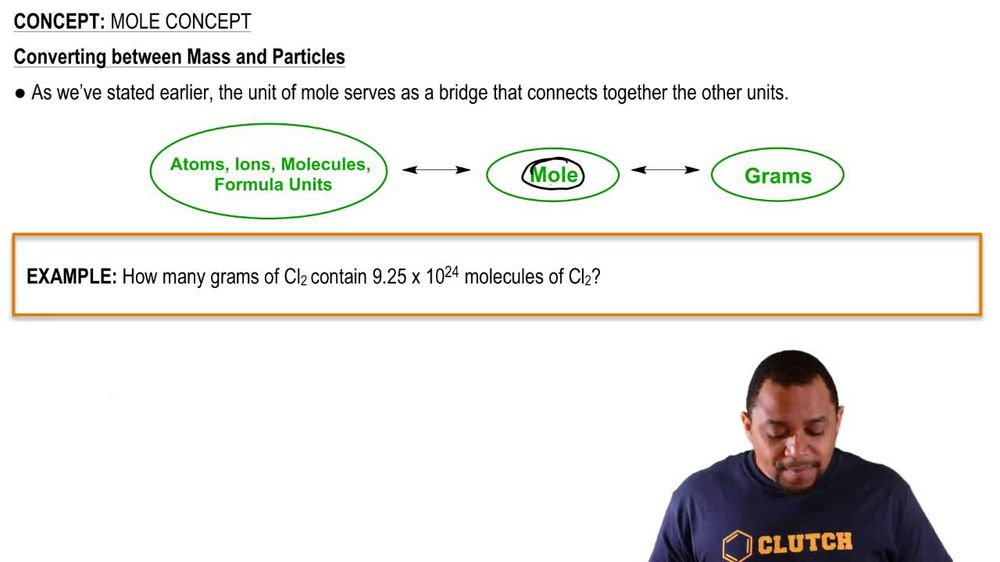Start typing, then use the up and down arrows to select an option from the list.
1. 2. Atoms & Elements2. Mole Concept
Problem

# Calculate mass (in grams) of sodium in 8.5 g of each sodiumcontaining food additive. a. NaCl (table salt)

Relevant Solution3m
Play a video:
Hey everyone welcome back. So let's get started with this video here. They want us to calculate the mass of nitrogen g and a 15.5 g sample of the nitrogen Penta oxide. Okay. So then Dina Tre Champion tox side that is going to be die. That means to nitrogen Penta oxide. That means five oxygen's okay. And then we saw for the molar mass And we get that the molar mass of nitrogen Penta oxide is 108 point 01 grams per mole. Okay. And we're starting with the given of grams. So from grams we're going to want to go to moles and how do we go to most? That is by using molar mass, which we said was 108 point 01 g per mole. Okay. But we don't want molds. We want the mass of nitrogen and grams in this sample. Therefore, we're going to go to moles of nitrogen. Once again, we want the mess. So finally we're going to go two g of nitrogen because that is the mass of nitrogen. So how do we go from Mosul nitrogen grams of nitrogen? We're going to use the molar mass of nitrogen. Just nitrogen. And we can find that on the periodic table which is point 007 g per mole. So let's go ahead and start with our store Akiyama tree. And we're going to start with the given, Which is 15.5 grams of sample of di nitrogen Penta oxide. So N 205. Okay. So from gramps you're going to go to most by using the molar mass. So then there is 108 .01g of end to 05. And one more of N 205. Okay, So then now we're going to go to most of nitrogen. So then one more of N contains two moles of nitrogen. Okay? So then now we're going to go from moles of nitrogen, two g of nitrogen. So then one more of nitrogen. And we're going to use a molar mass of just nitrogen, which is .007g of nitrogen For one mole of nitrogen. So then grams of N 205, cancel out moles of N 205, cancel out most of end cancel out. And we just get grams of end the mass of nitrogen. We suffer it and we get four 0. grams nitrogen. Okay, Thank you for watching. I hope this helped. And I'll see you in the next video.